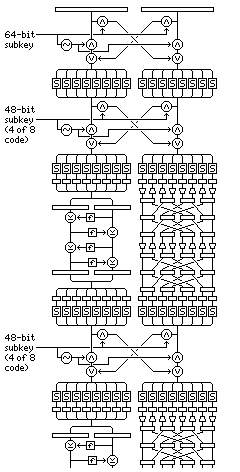[Next] [Up] [Previous] [Index]

# Combining Two Unrelated Block Ciphers

Another way of making use of the strength that can be obtained by using two block ciphers of a completely different type is illustrated below:Essentially, each round of encryption consists of applying four rounds of DES to the left half of the block, and two rounds of SAFER to the right half of the block. Six rounds of encryption are used, alternating with seven stages in which the left and right halves of the block are combined. (Even four rounds of encryption may be adequate.)

These fencing stages consist of applying a key-dependent S-box (whose inverse will be required for decryption) to the bytes of the block, and then swapping bits between halves by using a mask to indicate which bits are to be swapped with their corresponding bits in the other halves. This method was pioneered in the block cipher ICE.

To ensure that each bit being encrypted is evenly divided between the two halves of the block by each fencing stage, a 48-bit subkey, expanded by the use of a 4 of 8 code (as seen in the definition of QUADIBLOC) is used, so that exactly four bits of each byte are swapped.

An additional ICE-style swap is used at the beginning of the cipher, so that the use of byte substitution for whitening is not reduced in effectiveness by the use of the S-box at the start of the cipher. This swap uses a plain 64-bit subkey for maximum randomness, since equal division between halves does not serve a purpose in that position.

The intent of this design is, of course, that since two completely different ciphers are intimately mixed, analysis to find a weakness is essentially impossible.

For generating the key schedule, both the 48-bit keys for the fencing stages and the 48-bit DES subkeys are most easily generated in units six bits long; thus, if a source of bytes is used to produce the subkeys, it might be quickest to take only the least significant bits of eight bytes to form one of those 48-bit keys.

[Next] [Up] [Previous] [Index]